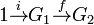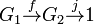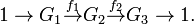# Exact sequence

In mathematics, particularly in abstract algebra and homological algebra, an exact sequence is a sequence of algebraic objects and morphisms which is used to describe or analyse algebraic structure. (See below for the formal definition.)

In general the concept of an exact sequence makes sense when dealing with algebraic structures for which there are the concepts of "objects", "morphisms" between objects and of "subobjects" attached to morphisms which play the role of "kernel" and "image".

We shall expound the concept in group theory: very similar remarks apply to module theory.

A sequence will simply be a collection of group homomorphisms fi and groups Gi with$G_{i-1} \stackrel{f_i}{\rightarrow} G_i$, indexed by some interval of the integers. A sequence will be termed exact at the term Gi if there are maps fi − 1 and fi to the left and right of the term Gi and the condition that the kernel of fi is equal to the image of fi − 1 holds. An exact sequence is one which is exact at every term at which the condition makes sense.

Exactness can be used to unify several concepts in group theory. For example, the assertion that the sequence$1 \stackrel{i}{\rightarrow} G_1 \stackrel{f}{\rightarrow} G_2 \,$

is exact asserts that f is injective. We see this by noting that the only possible homomorphism i from the trivial group has as image the trivial subgroup of G1 consisting of the identity, and the exactness condition is thus that the kernel of f is equal to this trivial subgroup, which is equivalent to the statement that f is injective.

Similarly, the assertion that the sequence$G_1 \stackrel{f}{\rightarrow} G_2 \stackrel{j}{\rightarrow} 1\,$

is exact asserts that f is surjective. We see this by noting that the only possible homomorphism j to the trivial group has as kernel the whole of G2, and the exactness condition is thus that the image of f is equal to this group, which is equivalent to the statement that f is surjective.

Combining these, exactness of$1 \rightarrow G_1 \stackrel{f}{\rightarrow} G_2 \rightarrow 1\,$

asserts that f is an isomorphism.

A short exact sequence is one of the form$1 \rightarrow G_1 \stackrel{f_1}{\rightarrow} G_2 \stackrel{f_2}{\rightarrow} G_3 \rightarrow 1 . \,$

It expresses the condition that G3 is the quotient of G2 by a subgroup isomorphic to G1: this may be expressed as saying that G2 is an extension of G3 by G1.Some content on this page may previously have appeared on Citizendium.